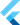# Deriving values from elements: fold, reduce, join

on Monday, 20th of July, 2020

`fold`, `reduce` and `join` are some of the methods on iterables and lists that are used to generate a single value by combining on the elements of the list.

`fold` and `reduce` are similar. They both iterate over the elements and perform an operation on each element and the element created thus far in the iteration. That's a wonky statement, so consider `reduce` first with this example:

``````  List<int> nums = [1, 2, 3, 4, 5];
final sum = nums.reduce((int sumSoFar, int currentInt) {
return sumSoFar + currentInt;
});``````

This example will add each number from the list together. Based on the variable names, perhaps you can see that at each iteration you have access to the reduced value (in this case the sum), so far, as well as the current element that is going to be added to the `sumSoFar`.

Running this in DartPad with print statements might help:

```    ```
void main() {
List nums = [1, 2, 3, 4, 5];
final sum = nums.reduce((int sumSoFar, int currentElement) {
print("sumSoFar \$sumSoFar");
print("currentElement \$currentElement");
print('--');
return sumSoFar + currentElement;
});
print("final sum == \$sum");
}
``````

As I mentioned, `fold` is quite similar to this. Before we look at fold, let's talk about the difference. `reduce` must return, from each iteration of the callback being called, the same type as the type of the elements of the list. for example:

`<int>[1,2,3].reduce(callback)` must return an int from each call to teh callback. `<String>["hello","world"].reduce(callback)` must return an `String` from each call to teh callback.

which means, this will throw an error:

``````void main() {
List<int> nums = [1, 2, 3, 4, 5];
final join = nums.reduce((int sumSoFar, int currentElement) {
return "\$sumSoFar\$currentElement"; // error! must return an int
});
}``````

#### fold

`fold` is essentially the solution to the issue above. You can derive values of different types from `fold`, but it behaves much in the same way that `reduce` does.

The API difference is in what values are passed to `fold`, and the callback passed to `fold`. `fold` needs a "starting value", which it uses in the first iteration of the callback.

```    ```
void main() {
List nums = [1, 2, 3, 4, 5];
// the starting value is an empty string.
// the values passed in are the derived value so far, as well as the current element.
final join = nums.fold("", (String stringSoFar, int currentElement) {
return "\$sumSoFar\$currentElement";
});
}
``````

`fold` is another method that I find myself using quite often in the day to day.